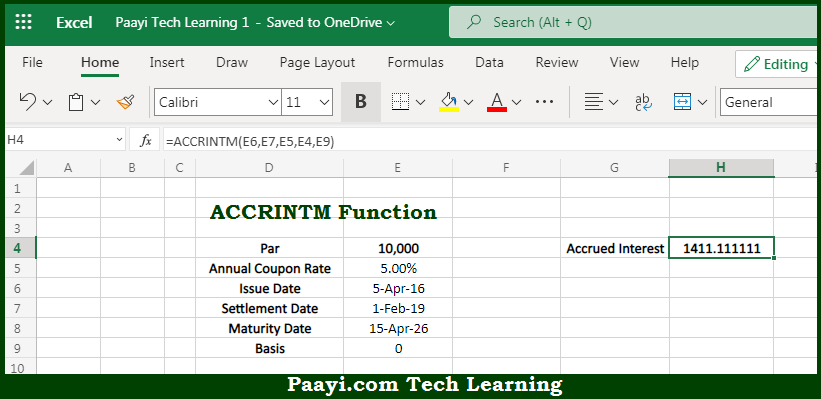# Learn How to Use Microsoft Excel ACCRINTM Function

Written by | 0 Comments | 707 Views

In this article, you will learn how to use the Microsoft Excel ACCRINTM function and its prime function in Microsoft Excel. You will also get to know the Microsoft Excel ACCRINTM function return value and syntax with the help of some examples.

Microsoft Excel ACCRINTM Function

The main purpose of the Microsoft Excel ACCRINTM function is to get the accrued interest at maturity. That implies, with the help of the ACCRINTM function you can able to return the accrued interest for a security that pays interest at maturity which means it pays interest one time only. So, with the help of the ACCRINTM function, you can able to get accrued interest at maturity.

Return Value of ACCRINTM Function

The return value will be the accrued interest.

Syntax of ACCRINTM Function

=ACCRINTM(id, sd, rate, par, [basis])

Where the arguments:

• id: This is the issue date of the security.
• sd: This is the settlement date of the security.
• rate: This is the annual coupon rate.
• par: This is the par value of the security.
• basis: This is the day count basis, default is 0 (optional).

How to Use Microsoft Excel ACCRINTM Function?So we know that Microsoft Excel ACCRINTM function you can able to get the accrued interest at the maturity. That implies, with the help of the ACCRINTM function you can able to return the accrued interest for a security that pays interest at maturity which means it pays interest one time only. So, with the help of the ACCRINTM function, you can able to get accrued interest at maturity.